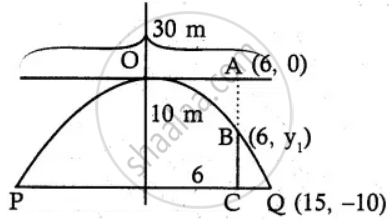Tamil Nadu Board of Secondary EducationHSC Arts Class 12th

# A bridge has a parabolic arch that is 10 m high in the centre and 30 m wide at the bottom. Find the height of the arch 6m from the centre, on either sides - Mathematics

Sum

A bridge has a parabolic arch that is 10 m high in the centre and 30 m wide at the bottom. Find the height of the arch 6m from the centre, on either sides

#### SolutionPQ = 2a = 30 m

a = 15 m

Point Q be (15 , –10)

Equation of the parabola

x2 = – 4 ay  .......(1)

Q lies on parabola

152 = – 4a( –10)

a = 225/40

(1) ⇒ x2 = - 4(225/40)y

x2 = 225/10 y

Let B(6, y) lies on parabola

62 = 225/10 y_1

y1 = - (36 xx 10)225

= (- 8)5

= 8/5 "m"

AB = AC – BC

= 10 - 8/5

= (50 - 8)/5

= 42/5

AB = 8.4 m

∴ The height of the arch 6 m from the centre is 8.4 m

Concept: Real Life Applications of Conics
Is there an error in this question or solution?

#### APPEARS IN

Tamil Nadu Board Samacheer Kalvi Class 12th Mathematics Volume 1 and 2 Answers Guide
Chapter 5 Two Dimensional Analytical Geometry-II
Exercise 5.5 | Q 1 | Page 214
Share